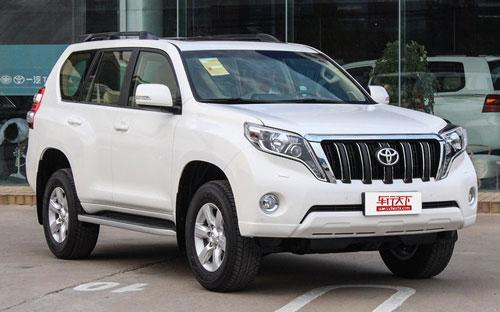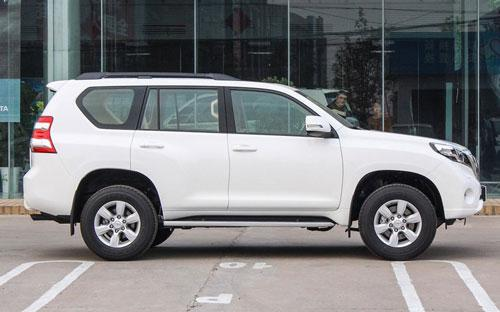一汽丰田 普拉多8 种颜色可选2016款最低售价：36.98 万元起

4780(mm)1885(mm)1845(mm)配置亮点：
• 胎压监测装置

• ISOFIX儿童座椅接口

• 车身稳定控制(ESC/ESP/DSC等)

• 电动天窗

• 定速巡航

• 后倒车雷达

• 真皮座椅

• GPS导航系统

• 氙气大灯

• 后视镜加热

2016款 3.5L 自动TX-L NAVI (177张)
• 2016款 3.5L 自动TX-L NAVI (177张)
• 2016款 2.7L 自动标准版 (150张)
• 2016款 2.7L 自动豪华版 (136张)
• 2016款 3.5L 自动TX-L (113张)一汽丰田 普拉多 经销商

查看更多 >>
更多 >>

本地降价排名

一汽丰田 普拉多 动力加速

普拉多 0-100公里加速时间分布在 0.0--秒 属于 超跑级

动力级别 加速时间 车型

一汽丰田 普拉多 视频

一汽丰田 普拉多 新闻资讯

国产新款普拉多上市 售价36.98-62.53万

上市新车 超过9223次关注

2015年9月24日晚，一汽丰田官方宣布旗下新款普拉多车型正式上市，此次推出的3.5L和2.7L车型，其中3.5L车型将取代此前在售的4.0L普拉多。新车共计推出八款细分车型，...

初春自驾出游 选牧马人还是普拉多

试驾评测 超过9425次关注

几处早莺争暖树，谁家新燕啄春泥，春意渐浓，想要选择一辆出游的工具，必须要一辆兼具公路性和四驱性的SUV才行，Jeep牧马人和丰田普拉多都是非常不错的好选择，到底...

国产普拉多3.5L

即将上市 超过11202次关注

国产普拉多3.5L车型将于9月24日正式上市，未来3.5L车型将取代现有的4.0L车型，届时国产普拉多将有2.7L和3.5L两款发动机供大家选择。

丰田将于2015年在中国投产2.7L普拉多

车系文章 超过3102次关注

前不久四川一汽丰田汽车有限公司成都工厂举行了动工仪式。这间工厂为中国第一汽车集团公司与丰田的合资生产企业。据了解，其于2015年3月开始正式生产丰田普拉多2.7L车型。

国产新款丰田普拉多价格公布 售53万元起

车系文章 超过3270次关注

丰田官网正式公布了旗下国产全新2014款丰田普拉多全系的官方指导价格，全系指导价格53万-67.6万元，相比老款车型，新款普拉多除VX配置车型价格小幅上涨外，其余车型...

适合长途出游SUV车型推荐 丰田普拉多

车系文章 超过4093次关注

用于长途旅行，在50-80万的级别的车型中绝对不能不提普拉多。倒不是说它的越野性能有多么强悍（不过确实很强悍），内饰有多么豪华，而是它的性价比是最高的，同时也...

各级别硬派越野车推荐 一汽丰田普拉多

车系文章 超过5382次关注

普拉多也是硬派越野的代表车型之一，和兰德酷路泽组成了丰田越野车的高低搭配，并且两款车的市场表现都足够出色。不同于很多同级别车型，普拉多在兼具强大通过性的...

换装霸气前脸 广州车展实拍丰田新普拉多

车系文章 超过4766次关注

丰田新款普拉多在本次广州车展中如约亮相，相比老款车型，新车主要对外观以及内部配置进行了升级，整体造型变得更霸气、更有力量感。新款普拉多2.7L车型还是会以进...

广汽丰田版“普拉多”将上市 售价30万起

车系文章 超过3158次关注

此前丰田在海外正式公布了其定位在中大型SUV市场的新款4Runner产品，并且该款车型也在国内进行了专利申报。目前广汽丰田在国内空间巨大的SUV市场仅有汉兰达一款产品...

猜你喜欢

﻿
• 快速找车
• 选择品牌
• 选择品牌
• A  奥迪
• A  阿斯顿·马丁
• A  阿尔法·罗密欧
• B  宝沃
• B  布加迪
• B  巴博斯
• B  保时捷
• B  宾利
• B  奔驰
• B  宝马
• B  本田
• B  别克
• B  标致
• B  比亚迪
• B  宝骏
• B  北汽制造
• B  北汽新能源
• B  北汽幻速
• B  北汽威旺
• B  北京汽车
• B  奔腾
• B  北汽绅宝
• C  长安
• C  长安商用
• C  长城
• C  昌河
• D  大众
• D  道奇
• D  DS
• D  东南
• D  东风风神
• D  东风风行
• D  东风小康
• D  东风风度
• D  东风
• F  福特
• F  丰田
• F  菲亚特
• F  法拉利
• F  福田
• F  福迪
• F  福汽启腾
• G  观致
• G  广汽传祺
• G  广汽吉奥
• G  GMC
• H  红旗
• H  汉腾汽车
• H  哈弗
• H  哈飞
• H  海格
• H  海马
• H  华颂
• H  黄海
• H  华泰
• H  恒天
• J  吉利汽车
• J  捷豹
• J  Jeep
• J  江淮
• J  江铃
• J  金杯
• J  九龙
• J  金旅
• K  凯翼
• K  凯迪拉克
• K  克莱斯勒
• K  科尼塞克
• K  卡威
• K  开瑞
• L  路虎
• L  林肯
• L  劳斯莱斯
• L  兰博基尼
• L  雷克萨斯
• L  铃木
• L  雷诺
• L  理念
• L  力帆
• L  莲花汽车
• L  猎豹
• L  路特斯
• L  陆风
• M  马自达
• M  MG
• M  MINI
• M  玛莎拉蒂
• M  摩根
• M  迈凯轮
• N  纳智捷
• O  欧宝
• O  讴歌
• O  欧朗
• Q  奇瑞
• Q  起亚
• Q  启辰
• R  日产
• R  荣威
• R  瑞麒
• S  三菱
• S  斯威汽车
• S  萨博
• S  smart
• S  斯柯达
• S  斯巴鲁
• S  思铭
• S  双龙
• S  上汽大通
• S  双环
• T  特斯拉
• T  腾势
• W  沃尔沃
• W  五菱汽车
• W  五十铃
• W  威兹曼
• W  威麟
• X  现代
• X  雪佛兰
• X  雪铁龙
• X  西雅特
• Y  一汽
• Y  英菲尼迪
• Y  英致
• Y  依维柯
• Y  野马汽车
• Y  永源
• Z  众泰
• Z  中华
• Z  中兴
• Z  知豆
• 选择车系
• 选择车系
• 车型对比
• 选择品牌
• 选择品牌
• A  奥迪
• A  阿斯顿·马丁
• A  阿尔法·罗密欧
• B  宝沃
• B  布加迪
• B  巴博斯
• B  保时捷
• B  宾利
• B  奔驰
• B  宝马
• B  本田
• B  别克
• B  标致
• B  比亚迪
• B  宝骏
• B  北汽制造
• B  北汽新能源
• B  北汽幻速
• B  北汽威旺
• B  北京汽车
• B  奔腾
• B  北汽绅宝
• C  长安
• C  长安商用
• C  长城
• C  昌河
• D  大众
• D  道奇
• D  DS
• D  东南
• D  东风风神
• D  东风风行
• D  东风小康
• D  东风风度
• D  东风
• F  福特
• F  丰田
• F  菲亚特
• F  法拉利
• F  福田
• F  福迪
• F  福汽启腾
• G  观致
• G  广汽传祺
• G  广汽吉奥
• G  GMC
• H  红旗
• H  汉腾汽车
• H  哈弗
• H  哈飞
• H  海格
• H  海马
• H  华颂
• H  黄海
• H  华泰
• H  恒天
• J  吉利汽车
• J  捷豹
• J  Jeep
• J  江淮
• J  江铃
• J  金杯
• J  九龙
• J  金旅
• K  凯翼
• K  凯迪拉克
• K  克莱斯勒
• K  科尼塞克
• K  卡威
• K  开瑞
• L  路虎
• L  林肯
• L  劳斯莱斯
• L  兰博基尼
• L  雷克萨斯
• L  铃木
• L  雷诺
• L  理念
• L  力帆
• L  莲花汽车
• L  猎豹
• L  路特斯
• L  陆风
• M  马自达
• M  MG
• M  MINI
• M  玛莎拉蒂
• M  摩根
• M  迈凯轮
• N  纳智捷
• O  欧宝
• O  讴歌
• O  欧朗
• Q  奇瑞
• Q  起亚
• Q  启辰
• R  日产
• R  荣威
• R  瑞麒
• S  三菱
• S  斯威汽车
• S  萨博
• S  smart
• S  斯柯达
• S  斯巴鲁
• S  思铭
• S  双龙
• S  上汽大通
• S  双环
• T  特斯拉
• T  腾势
• W  沃尔沃
• W  五菱汽车
• W  五十铃
• W  威兹曼
• W  威麟
• X  现代
• X  雪佛兰
• X  雪铁龙
• X  西雅特
• Y  一汽
• Y  英菲尼迪
• Y  英致
• Y  依维柯
• Y  野马汽车
• Y  永源
• Z  众泰
• Z  中华
• Z  中兴
• Z  知豆
• 选择车系
• 选择车系
• 选择车型
• 选择车型
• 意见反馈